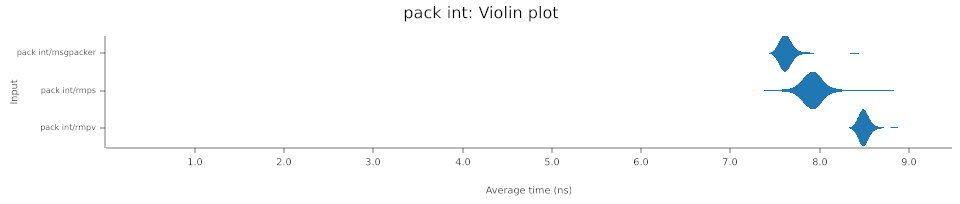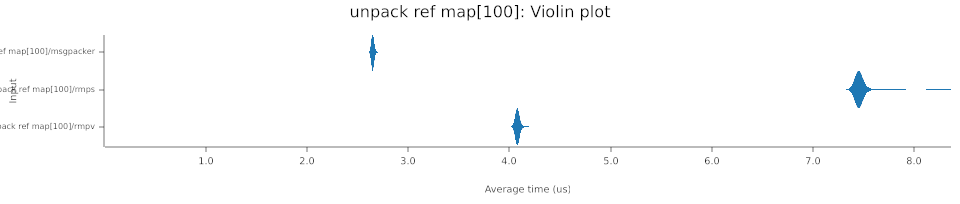# Crate msgpacker[−][src]

Expand description

## MessagePacker - some Rust in the msgpack protocol

The protocol specification can be found here.

This crate targets simplicity and performance. No dependencies are used, just the standard Rust library.

We have two main structures available:

• Message - Owned parsed values
• MessageRef - Message parsed by reference and bound to the lifetime of the readers source

### Example

``````use msgpacker::prelude::*;
use std::io::{Cursor, Seek};

let buffer = vec![0u8; 4096];
let mut cursor = Cursor::new(buffer);

let key = Message::string("some-key");
let value = Message::integer_signed(-15);
let entry = MapEntry::new(key, value);
let message = Message::map(vec![entry]);

// Write the message to the cursor
message.pack(&mut cursor).expect("Message pack failed");

cursor.rewind().expect("Reset the cursor to the beginning");

// Read the message from the cursor
let restored = Message::unpack(&mut cursor).expect("Message unpack failed");
let value = restored
.as_map()
.expect("A map was originally created")
.first()
.expect("The map contained one entry")
.val()
.as_integer()
.expect("The value was an integer")
.as_i64()
.expect("The value was a negative integer");

assert_eq!(value, -15);

// Alternatively, we can use the index implementation
let value = restored["some-key"]
.as_integer()
.expect("The value was an integer")
.as_i64()
.expect("The value was a negative number");

assert_eq!(value, -15);``````

### Example (by ref)

``````use msgpacker::prelude::*;
use std::io::{Cursor, Seek};

let mut cursor = Cursor::new(vec![0u8; 4096]);

let key = Message::String("some-key".into());
let value = Message::Integer(Integer::signed(-15));
let entry = MapEntry::new(key, value);
let message = Message::Map(vec![entry]);

// Write the message to the cursor
message.pack(&mut cursor).expect("Message pack failed");

cursor.rewind().expect("Reset the cursor to the beginning");

// The consumer need to guarantee himself the cursor source will live long enough to satisfy the
// lifetime of the message reference.
//
// If this is guaranteed, then the function is safe.
let restored = unsafe { MessageRef::unpack(&mut cursor).expect("Message unpack failed") };

// The lifetime of `MessageRef` is not bound to the `Read` implementation because the source
// might outlive it - as in this example
let _buffer = cursor.into_inner();

// `MessageRef` behaves the same as `Message`, but the runtime cost is cheaper because it will
// avoid a couple of unnecessary copies
let value = restored
.as_map()
.expect("A map was originally created")
.first()
.expect("The map contained one entry")
.val()
.as_integer()
.expect("The value was an integer")
.as_i64()
.expect("The value was a negative integer");

assert_eq!(value, -15);

// MessageRef also implements `Index`
let value = restored["some-key"]
.as_integer()
.expect("The value was an integer")
.as_i64()
.expect("The value was a negative number");

assert_eq!(value, -15);``````

### Benchmarks

Results obtained with `Intel(R) Core(TM) i9-9900X CPU @ 3.50GHz`. To generate benchmarks, run `\$ cargo bench`.

The benchmark compares msgpacker with two very popuplar Rust implementations: rmpv and rmps. The performance was similar for pack and unpack, with msgpacker taking the lead a couple of times. Very often rmps was far behind.

The performance of integer packing was better for msgpacker.However, for unpack by reference, the performance was dramatically better in favor of msgpacker for map deserialization.The full report can be found here.

## Modules

Prelude containing all public types of the crate

Internal types of the message

## Enums

MessagePack protocol type

MessagePack protocol type used as reference.